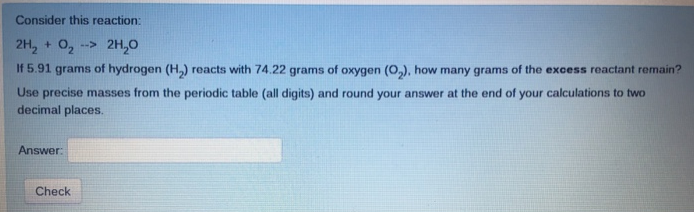# Problem: Consider this reaction 2H2 + O2 → 2H2O If 5.91 grams of hydrogen (H2) reacts with 74.22 grams of oxygen (O2), how many grams of the excess reactant remain? Use precise masses from the periodic table (all digits) and round your answer at the end of your calculations to two decimal places.

###### FREE Expert Solution
97% (107 ratings)###### Problem Details

Consider this reaction

2H2 + O2 → 2H2

If 5.91 grams of hydrogen (H2) reacts with 74.22 grams of oxygen (O2), how many grams of the excess reactant remain? Use precise masses from the periodic table (all digits) and round your answer at the end of your calculations to two decimal places.